# G-2: Path Dependent

In this lesson, we will continue to learn about the tools and features of GeoGebra. Where possible, we will do this by working on geometrically interesting constructions. Hopefully this will give you examples and insight on how you might use GeoGebra within your teaching practice.

## Points on Objects

So far, most of the points we have created have been Free Objects. Now we create points with restrictions.

Create a circle using the tool of your choice, then select the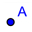New Point tool. When you move the mouse over the circle, you will notice that the circle becomes highlighted. Click on the circle, then select the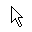Move tool. Try dragging the newly created point around. It’s stuck to the circle! We will call this kind of point a Path Dependent point. Path dependent points can also be created on lines, line segments, arcs, conics, and any other path like object.

Points can also be created within a region using the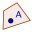Point on Object tool. Note that in order to restrict a point to, for example, the interior of a circle, you need to first increase the Opacity of the object from 0 in the Object Properties.

Terminology

In GeoGebra, you will not see the term Path Dependent. In general, GeoGebra uses Point on Object to describe either a point on a path or a point in a region. We introduced this terminology here to make it explicit that we are referring to a point on a path (as opposed to a point in a region, or an intersection point, etc.), as some of the tools below require a Path Dependent point.

### Construction: Angles subtended by an arc in a circle

This exercise will demonstrate properties of angles within a circle. Create a circle with center ‘A’ through a point ‘B’. Once you have adjusted the circle to your liking, hide ‘A’ and ‘B’. Use the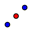Midpoint or Center tool to recreate the center of your circle, labelled ‘C’. Then create a chord in the circle using two path dependent points ‘D’ and ‘E’.

Well-Designed Constructions

Why do we need to create two new points to make a chord? Could we not use ‘B’ as one of our points of the chord? We could, but the radius of the circle is dependent on ‘B’. By creating two points strictly dependent on the circle itself, it will be easier to separate properties of the circle from the defining parameters of the circle. This is also the reasoning behind creating a new center, as we will use the center later.

Do not feel that you must do this for every construction. In fact, it may make sense to use the original points used to construct the object depending on the situation. Use your judgement in future exercises as to whether these kinds of precautions are necessary.

Our next step is to create a point on the circle that is restricted to one of the two arcs created by ‘D’ and ‘E’. To do this, first hide the circle. Then locate the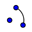Circular Arc with Center between Two Points tool. Select ‘C’, ‘D’, then ‘E’. You should then have an arc traveling counter-clockwise from ‘D’ to ‘E’. Create a point ‘F’ on this arc. Hide the arc and show the original circle.

Construct segments CD, CE, FD and FE. Locate the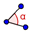Angle tool. To calculate the angles at ‘C’ and ‘F’ using the three point method (as opposed to the two line method), select ‘E’, the vertex, then ‘D’. By default, the angle will be measured counter-clockwise (though you can change this in the angle’s object properties), so had we chosen ‘D’ first, we would have measured the reflex angle at the vertex.

Now feel free to move the path dependent points one at a time and see what properties hold in this construction. What do we know about $$\angle$$ECD when DE is a diameter of the circle?

### Multiple Intersections

In lesson G-0, you were asked to find an intersection of two lines. There are two methods for finding the intersection of intersecting paths. It is possible that you used the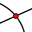Intersect Two Objects tool. Another method is to use theNew Point tool. With this tool selected, move the mouse over two intersecting paths. You should see both paths highlighted. Click, and you will now have a point residing at the intersection of the two paths.

Note that you will not be able to drag this point around like other Free Objects or Path Dependent points, nor will you be able to move objects created using this point (try using an intersection point as the center of a circle).

Try using both methods to find the intersection of a line and a circle. What is the difference in what the two methods do?

As a guideline, you may want to use the Intersect Two Objects tool if:

• There are 3 or more paths intersecting at one point. GeoGebra is not able to over-specify how many paths are used in an intersection (it must always be 2). In these cases, choose two of the paths to create the intersection.
• There are other objects near the intersection. You may not be able to select the intersection with the New Point tool if the other object is too close.

Also, be cautious when using the New Point tool. If you move at all when you click, you may only create a Path Dependent point on one path instead of an intersection. You will know this by the color of the resulting point.

### Construction: Falling Ladder Problem

Problem: There is a 4 metre ladder resting against the wall of a house. Dave is standing at the midpoint of the ladder when the base begins to slip outward. Create a construction to model this, and describe the path that Dave follows.

Solution: Create points ‘A’, ‘B’ and ‘C’ at points (0,0), (4,0) and (0,4) respectively, and then hide the axes. Draw segments AB and AC. These two segments will act as our wall and ground.

Create a point ‘D’ on segment AB. Then draw a circle centered at ‘D’ with radius 4. Construct the intersection ‘E’ of the circle and segment, then hide the circle. Then the segment DE represent the path of the ladder as ‘D’ moves from ‘A’ to ‘B’, and the midpoint ‘F’ of DE represents Dave’s position.

In order to visualize the path of the midpoint, we have two options. We can trace the path of ‘F’ by right-clicking (on Mac, hold ctrl and click) and turning trace on. Then as you move ‘D’, F will leave a trail. Once you have experimented with this, turn the trace off by right-clicking and choosing the same option.

Alternatively, we can use the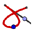Locus tool. With this tool selected, first click ‘F’, then click ‘D’. This will draw the path that ‘F’ will follow as ‘D’ is moved along the segment. By inspection, we determine that the path Dave follows when the ladder falls is a circular path.

If the Locus tool can be used to find the path of an object, it is generally a better solution than tracing the object. If we decided to move the entire construction all at once, trace would leave a mark as we moved it across the screen. However, the Locus tool only shows the path that occurs when ‘D’ is moved. In other words, it shows at any given time the path of ‘F’ given that everything but ‘D’ remains the same.

If you think you have a situation where the Locus tool could be used, but are not getting any objects appearing when using the tool, make sure you adhere to the following conditions:

• The second point given to Locus must be either a Path Dependent point, or a slider (sliders covered next lesson)
• The first point given to Locus must somehow depend on the second point.

How GeoGebra sees Locus

It’s important to note that GeoGebra does not actually store loci as a path. In more complicated diagrams, the locus may not be a recognized GeoGebra object, so GeoGebra really sees a locus as a collection of points very closely spaced.

For this reason, you cannot intersect a path with a locus and you cannot compare a path to a locus. You can check to see if a point in on a locus, but this process is not reliable, as the point you are checking may not have been included in the list of points stored. The one thing you can do is create a Path Dependent point on the locus.

Exercise (nickname: seconddiff)

Complete the following construction and answer the question below. You will have to create some additional objects to carry out some of the steps (the details are up to you).

1. Create a point ‘A’ at (0,1).
2. Create a horizontal line ‘l’ (L lowercase) at y=-1.
3. Create a Path Dependent point ‘V’ on the line ‘l’.
4. Create the point ‘W’ for which:
• ‘W’ is on the line through ‘V’ perpendicular to ‘l’
• The distance VW is exactly equal to the distance AW. (Hint: all points equally distant from two points lie on a particular line.)
• (You need to construct W with geometric tools, so that both of the above two facts remain exactly true even if you move V later.)
5. Draw the locus of ‘W’ with respect to ‘V’.

By analyzing the diagram, determine the equation of the locus.  You do not need a formal proof, but you may want to test your answer by checking a few of the values that ‘W’ takes as you move ‘V’ along the line ‘l’.

To submit: Hide any additional objects used to help create the diagram; only the five objects listed above should be showing. To display the equation of the graph, use a text box. Use GeoGebra’s built-in LaTeX styling for displaying the text box contents; if the font styles look different from how LaTeX normally looks, feel free to change to the serif option in the Object Properties, but we will accept either format.

Note: Do not use conics to complete this exercise.

These are course notes for the University of Waterloo's course Math 600: Mathematical Software.
© 2012—. Written and developed by David Pritchard and Stephen Tosh. Contact (goes to the CEMC)